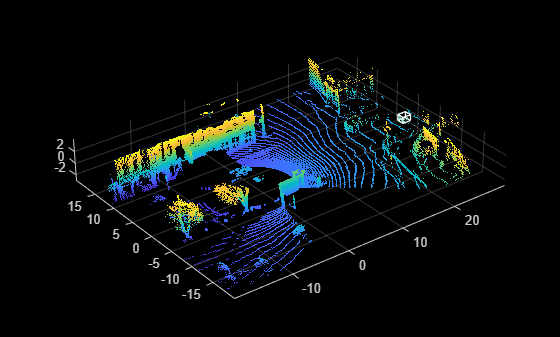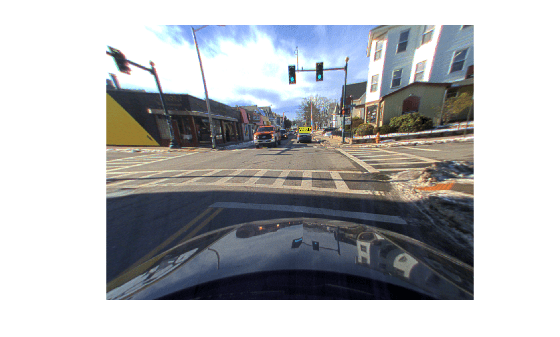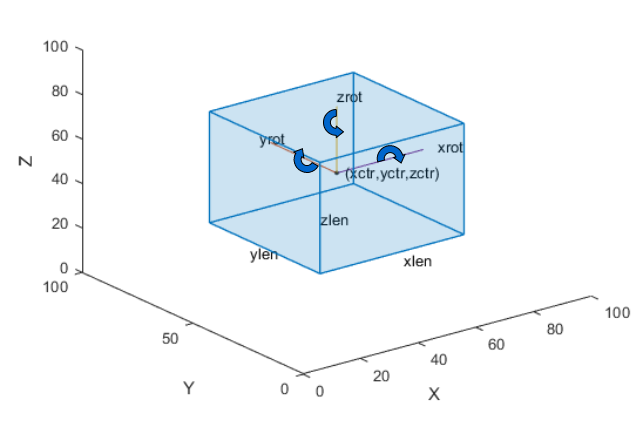Main Content

# bboxLidarToCamera

Estimate 2-D bounding box in camera frame using 3-D bounding box in lidar frame

## Syntax

``bboxesCamera = bboxLidarToCamera(bboxesLidar,intrinsics,tform)``
``bboxesCamera = bboxLidarToCamera(bboxesLidar,intrinsics,tform,L)``
``[bboxesCamera,boxesUsed] = bboxLidarToCamera(___)``
``[___] = bboxLidarToCamera(___,'ProjectedCuboid',true)``

## Description

````bboxesCamera = bboxLidarToCamera(bboxesLidar,intrinsics,tform)` estimates 2-D bounding boxes in the camera frame from 3-D bounding boxes in the lidar frame `bboxesLidar`. The function uses the camera intrinsic parameters `intrinsics` and a lidar to camera transformation matrix `tform`.```
````bboxesCamera = bboxLidarToCamera(bboxesLidar,intrinsics,tform,L)` further refines the 2-D bounding boxes to the edges of the object inside it using `L`. `L` is the corresponding labeled 2-D image of the 2-D bounding boxes, where the objects are labeled distinctively.```
````[bboxesCamera,boxesUsed] = bboxLidarToCamera(___)` indicates for which of the specified 3-D bounding boxes the function detects a corresponding 2-D bounding box in the camera frame.```

example

````[___] = bboxLidarToCamera(___,'ProjectedCuboid',true)` returns 3-D projected cuboids instead of 2-D bounding boxes.```

## Examples

collapse all

Load ground truth data from a MAT file into the workspace. Extract the image, point cloud data, and camera intrinsic parameters from the ground truth data.

```dataPath = fullfile(toolboxdir('lidar'),'lidardata','lcc','bboxGT.mat'); gt = load(dataPath); im = gt.im; pc = gt.pc; intrinsics = gt.cameraParams;```

Extract the lidar to camera transformation matrix from the ground truth data.

`tform = gt.camToLidar.invert;`

Extract the 3-D bounding box information.

`bboxLidar = gt.cuboid1;`

Estimate the 2-D bounding box on the image.

`bboxesCamera = bboxLidarToCamera(bboxLidar,intrinsics,tform);`

Display the 3-D bounding box overlaid on the point cloud.

```pcshow(pc.Location,pc.Location(:,1)) showShape('cuboid',bboxLidar)```Display the 2-D bounding box overlaid on the image.

```J = undistortImage(im,intrinsics); annotatedImage = insertObjectAnnotation(J,'Rectangle',bboxesCamera,'Vehicle'); imshow(annotatedImage)```Load ground truth data from a MAT file into the workspace. Extract the image, point cloud data, and camera intrinsic parameters from the ground truth data.

```dataPath = fullfile(toolboxdir('lidar'),'lidardata','lcc','bboxGT.mat'); gt = load(dataPath); im = gt.im; pc = gt.pc; intrinsics = gt.cameraParams;```

Extract the lidar to camera transformation matrix from the ground truth data.

`tform = gt.camToLidar.invert;`

Extract the 3-D bounding box information.

`bboxLidar = gt.cuboid2;`

Estimate the projected 3-D bounding box on the image.

```bboxesCamera = bboxLidarToCamera(bboxLidar,intrinsics,tform,... 'ProjectedCuboid',true);```

Display the 3-D bounding box overlaid on the point cloud.

```figure pcshow(pc.Location,pc.Location(:,1)) showShape('cuboid',bboxLidar)```Display the 3-D projected bounding box overlaid on the image.

```J = undistortImage(im,intrinsics); h = imshow(J); pcH = vision.roi.ProjectedCuboid; pcH.Parent = h.Parent; pcH.Position = bboxesCamera;```## Input Arguments

collapse all

3-D bounding boxes in the lidar frame, specified as a `cuboidModel` object or an N-by-9 matrix of real values. N is the number of 3-D bounding boxes. Each row of the matrix has the form [xctr yctr zctr xlen ylen zlen xrot yrot zrot].

• xctr, yctr, and zctr — These values specify the x-, y-, and z-axis coordinates, respectively, of the center of the cuboid bounding box.

• xlen, ylen, and zlen — These values specify the length of the cuboid along the x-, y-, and z-axis, respectively, before it is rotated.

• xrot, yrot, and zrot — These values specify the rotation angles of the cuboid around the x-, y-, and z-axis, respectively. These angles are clockwise-positive when you look in the forward direction of their corresponding axes.

This figure shows how these values determine the position of a cuboid.Note

The function assumes that the point cloud data that corresponds to the 3-D bounding boxes and the image data are time synchronized.

Data Types: `single` | `double`

Camera intrinsic parameters, specified as a `cameraIntrinsics` object.

Camera to lidar rigid transformation, specified as a `rigid3d` object.

Labeled 2-D image, specified as a matrix of real values. The matrix size is the same as the `ImageSize` property of `intrinsics`.

Note

Labeled images are assumed to be undistorted.

Data Types: `single` | `double` | `int8` | `int16` | `uint8` | `uint16`

## Output Arguments

collapse all

2-D bounding boxes in the camera frame, returned as an M-by-4 matrix of real values. M is the number of detected bounding boxes. Each row of the matrix contains the location and size of a rectangular bounding box in the form [x y width height]. The x and y elements specify the x and y coordinates, respectively, for the upper-left corner of the rectangle. The width and height elements specify the size of the rectangle.

If `'ProjectedCuboid'` is set to `true`, the 2-D bounding boxes are returned as an M-by-8 matrix of real values. The bounding boxes have a cuboid shape and enclose the object. Each row of the matrix contains the size and location of the cuboid bounding box in the form [frontFace backFace]. Both the faces are represented as 2-D bounding boxes.

Data Types: `single` | `double`

Bounding box detection flag, returned as an N-element row vector of logicals. 2 is the number of input 3-D bounding boxes. If the function detects a corresponding 2-D bounding box in the camera frame, then it returns a value of `true` for that input 3-D bounding box. If the function does not detect a corresponding 2-D bounding box, then it returns a value of `false`.

Data Types: `logical`

## See Also

### Functions

Introduced in R2021a

## SupportGet trial now